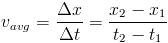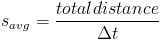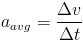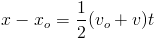# Summary of Chapter 2: Kinematics

Position: The Position of a particle is denoted by x, y or z depending which axis the particle is located on. Position is measured from the origin of the axis on which the particle is located.

Displacement: the distpacement of a particle (Δx) is the change in that particle's position. Displacement is a Vector quantity, it can be posative or negative.

Average Velocity:Velocity results from change in position over a change in time. So, the average velocity is given by:Velocity is a vector quantity, so it can be posative or negative. velocity is dependent on the total distance traveled, not the path by which that distance is traveled.

Average Speed Speed is similar to velocity, however it is not a vector quantity, it depends on the total distance traveled over a time interval.Average Acceleration Acceleration is the change in velocity over a certain time interval. Acceleration is also a vector quantity. Acceleration is given by:Constant Acceleration Under conditions of constant acceleration, five euqations apply to find the position, initial velocity final velocity, acceleration and time traveled by a particle. Each equation excludes one of the variables mentioned. These equations are listed below:

 Equation Excluded Variablex-xovvoat